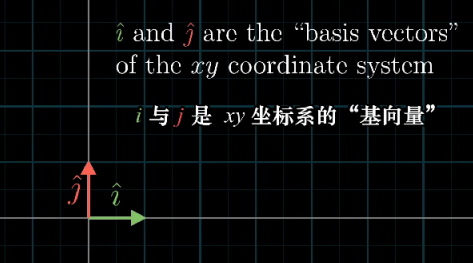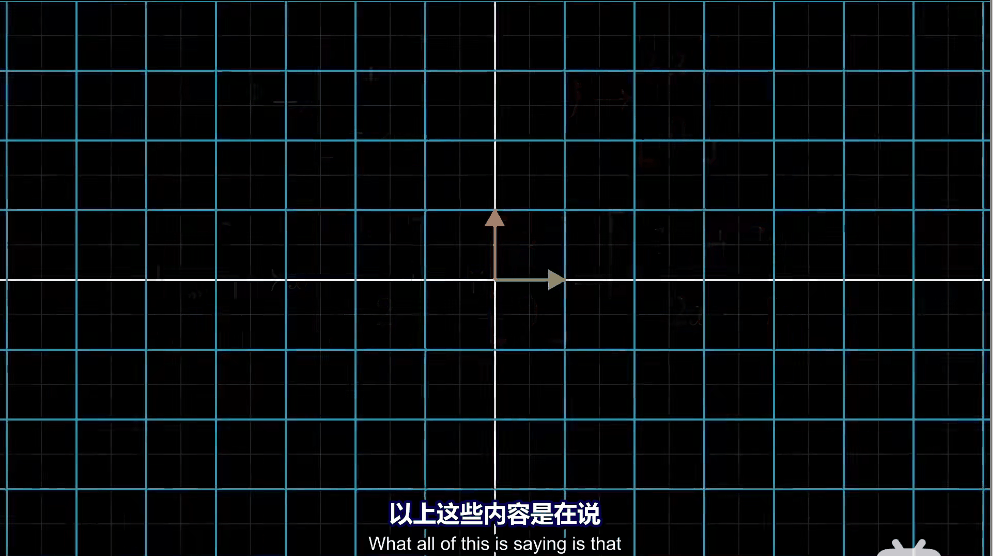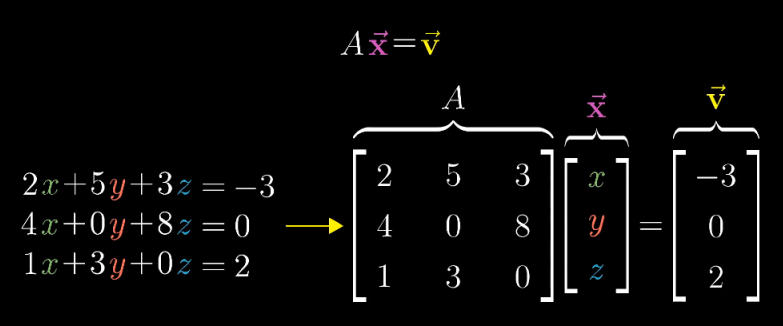# 线性代数自用超基础笔记

## 向量是什么？

$\begin{bmatrix}-2\\\\ 3\end{bmatrix}$是一个原点在$(0,0)$，终点在$(-2,3)$的箭头。

$\begin{bmatrix}2\\\\ 1\\\\ 3\end{bmatrix}$是一个原点在$(0,0,0)$，终点在$(2,1,3)$的箭头。

### 基向量## 矩阵乘法与线性变换（Linear Transformation）

“变换”类似于一个“函数”，输入进一个向量，输出一个向量，而用“变换”这一次的原因是需要我们从运动的角度看待，即输入向量运动到输出向量上。而考虑整个变换，则要考虑空间内所有向量经过变换后到达对应的另一个向量。$\begin{bmatrix}a&b\\\\ c&d\end{bmatrix}\begin{bmatrix}x\\\\ y\end{bmatrix}$

## 行列式

2x2的行列式很好算，就是左上乘右下减去右上成左下。

$def(\begin{bmatrix}3&2\\\\ 0&2\end{bmatrix})=6$

## 线性方程组与矩阵## 特征值 特征向量（Eigenvalue Eigenvector）

### 特征值分解

$A=P\Lambda P^{-1}$